• No products in the cart.

# Rate of Change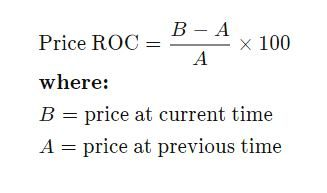## EXPRESSED GRAPHICALLY

A rate of change is a rate that describes how one quantity changes in relation to another quantity. If xx is the independent variable and yy is the dependent variable, then

rateofchange=changeinychangeinxrate  of  change=change  in  ychange  in  x

Rates of change can be positive or negative. This corresponds to an increase or decrease in the yy -value between the two data points. When a quantity does not change over time, it is called zero rate of change.

### Positive rate of change

When the value of xx increases, the value of yy increases and the graph slants upward.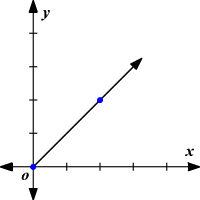### Negative rate of change

When the value of xx increases, the value of yy decreases and the graph slants downward.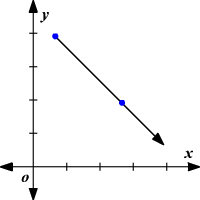### Zero rate of change

When the value of xx increases, the value of yy remains constant. That is, there is no change in yy value and the graph is a horizontal line .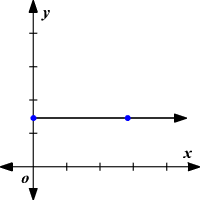Example:

Use the table to find the rate of change. Then graph it.

TimeDriving(h)x246DistanceTravelled(mi)y80160240Time  Driving  (h) xDistance  Travelled (mi) y28041606240

A rate of change is a rate that describes how one quantity changes in relation to another quantity.

rateofchange=changeinychangeinx=changeindistancechangeintime=1608042=802=401rate  of  change=change  in  ychange  in  x=change  in  distancechange  in  time=160−804−2=802=401

The rate of change is 401401 or 4040 . This means a vehicle is traveling at a rate of 4040 miles per hour.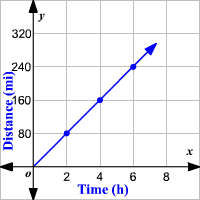Under this topic we shall consider the rates of change of
1. Distance with time
2. Velocity with time.
The rates of change may also be obtained from gradients of graphs, so we shall specifically consider
1. Distance – time graphs
2. Velocity – time graphs
A VIDEO BELOW ABOUT RATE OF CHANGE

1. DISTANCE – TIME GRAPHS
Example;
1. Tony walks 3 km at a constant speed, taking 50 minutes. He then runs 5 km at a constant speed, taking 25 minutes. Draw a distance time graphs of Tony Journey. Using a scale of 2 cm = 1 km and 1 m a scale of 2 cm = 1 km and 1 cm = 10 minutes. Use the graph to find his average speed for the whole journey in m/s.

2. Mary travels by car. At constant speed, from her home to London which is 70 km a way. She leaves home at 2.00 pm and arrives at 2.45 pm. She stays in London for 2 hours, then return homes , taking 50 minutes. Draw a distance – time graph showing Mary’s journey to and from London. Use a scale of 1 cm= 20 minutes and 2 cm = 10 km. Use the graph to find her velocity.
2. VELOCITY- TIME GRAPHS
From the velocity – time graphs we may obtain the distance covered by the body and the acceleration of the body.
Distance covered – Area under the curve.
Acceleration at a point – gradient of graph.
WAVES
THE VIDEO BELOW IS ABOUT DISTANCE TIME GRAPHS

# Unit CircleThe “Unit Circle” is a circle with a radius of 1.

Being so simple, it is a great way to learn and talk about lengths and angles.

The center is put on a graph where the x axis and y axis cross, so we get this neat arrangement here.## Sine, Cosine and Tangent

Because the radius is 1, we can directly measure sine, cosine and tangent.What happens when the angle, θ, is 0°?

cos 0° = 1, sin 0° = 0 and tan 0° = 0What happens when θ is 90°?

cos 90° = 0, sin 90° = 1 and tan 90° is undefined

Note ; The x- coordinate gives the cosine of the angle and the y- coordinate gives the sine of the angle , the coordinate can therefore be given in the form p(cosθ,Sin θ)
Where θ is the angle , the radius makes with the x-axis.
If the radius is multiplied by a scale 2, 3…..(Unit circle enlarged) then the coordinates are given in form of p(rcosθ,r sinθ)where r is 1,2,3…….

## Pythagoras

Pythagoras’ Theorem says that for a right angled triangle, the square of the long side equals the sum of the squares of the other two sides:

x2 + y2 = 12

But 12 is just 1, so:

x2 + y2 = 1
(the equation of the unit circle)

Also, since x=cos and y=sin, we get:

(cos(θ))2 + (sin(θ))2 = 1

a useful “identity”

## Important Angles: 30°, 45° and 60°

You should try to remember sin, cos and tan for the angles 30°, 45° and 60°.

Yes, yes, it is a pain to have to remember things, but it will make life easier when you know them, not just in exams, but other times when you need to do quick estimates, etc.

These are the values you should remember!

AngleSinCosTan=Sin/Cos
30°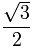1√3 = √33
45°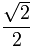1
60°√3

### How To Remember?sin(30°)  =  12  =  12  (because √1 = 1)

sin(45°)  =  22

sin(60°)  =  32

And cos goes “3,2,1”

cos(30°)  =  32

cos(45°)  =  22

cos(60°)  =  12  =  12

## Just 3 Numbers

In fact, knowing 3 numbers is enough: 12 ,  √22  and  √32

Because they work for both cos and sin: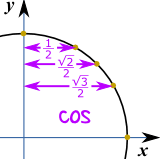Well, tan = sin/cos, so we can calculate it like this:

tan(30°) =sin(30°)cos(30°) = 1/2√3/2 = 1√3 = √33 *

tan(45°) =sin(45°)cos(45°) = √2/2√2/2 = 1

tan(60°) =sin(60°)cos(60°) = √3/21/2 = √3

* Note: writing 1√3 may cost you marks (see Rational Denominators), so instead use √33

## Quick Sketch

Another way to help you remember 30° and 60° is to make a quick sketch:

 Draw a triangle with side lengths of 2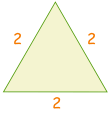Cut in half. Pythagoras says the new side is √312 + (√3)2 = 221 + 3 = 4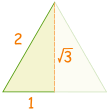Then use sohcahtoa for sin, cos or tan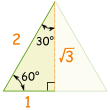### Example: sin(30°)

Sine: sohcahtoa

sine is opposite divided by hypotenuse
sin(30°) = oppositehypotenuse = 12## The Whole Circle

For the whole circle we need values in every quadrant, with the correct plus or minus sign as per Cartesian Coordinates:

Note that cos is first and sin is second, so it goes (cos, sin):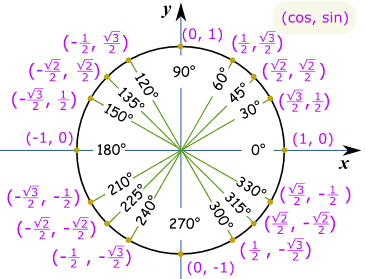Save as PDF

### Example: What is cos(330°) ?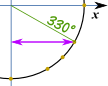Make a sketch like this, and we can see it is the “long” value:  √32

And this is the same Unit Circle in radians.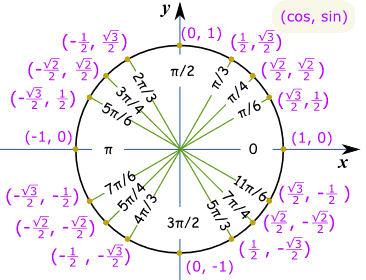### Example: What is sin(7π/6) ?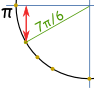Think “7π/6 = π + π/6″, then make a sketch.

We can then see it is negative and is the “short” value: −½

## What are Mean Median and Mode?

Mean, median and mode are all measures of central tendency in statistics. In different ways they each tell us what value in a data set is typical or representative of the data set.

The mean is the same as the average value of a data set and is found using a calculation. Add up all of the numbers and divide by the number of numbers in the data set.

The median is the central number of a data set. Arrange data points from smallest to largest and locate the central number. This is the median. If there are 2 numbers in the middle, the median is the average of those 2 numbers.

The mode is the number in a data set that occurs most frequently. Count how many times each number occurs in the data set. The mode is the number with the highest tally. It’s ok if there is more than one mode. And if all numbers occur the same number of times there is no mode.

## How to Find the Mean

1. Add up all data values to get the sum
2. Count the number of values in your data set
3. Divide the sum by the count

The mean is the same as the average value in a data set.

## Mean Formula

The mean  of a data set is the sum of all the data divided by the count n.

mean=x¯¯¯=ni=1xinmean=x¯=∑i=1nxin

## How to Find the Median

The median x˜x~ is the data value separating the upper half of a data set from the lower half.

• Arrange data values from lowest to highest value
• The median is the data value in the middle of the set
• If there are 2 data values in the middle the median is the mean of those 2 values.

## Median Example

For the data set 1, 1, 2, 5, 6, 6, 9 the median is 5.

For the data set 1, 1, 26, 6, 9 the median is 4. Take the mean of 2 and 6 or, (2+6)/2 = 4.

## Median Formula

Ordering a data set x1 ≤ x2 ≤ x3 ≤ … ≤ xn from lowest to highest value, the median x˜x~ is the data point separating the upper half of the data values from the lower half.

If the size of the data set n is odd the median is the value at position p where

p=n+12p=n+12
x˜=xpx~=xp

If n is even the median is the average of the values at positions p and p + 1 where

p=n2p=n2
x˜=xp+xp+12x~=xp+xp+12

## How to Find the Mode

Mode is the value or values in the data set that occur most frequently.

For the data set 11, 2, 5, 66, 9 the mode is 1 and also 6.

IQR = Q3  – Q1

## Outliers

Potential Outliers are values that lie above the Upper Fence or below the Lower Fence of the sample set.
Upper Fence = Q3 + 1.5 × Interquartile Range
Lower Fence = Q1 − 1.5 × Interquartile Range

A VIDEO BELOW SHOWING HOW TO SOLVE PROBLEMS WITH AVERAGE AND STATISTICS

Statistics: The average | Descriptive statistics | Probability and Statistics | Khan Academy

An example of a Quadratic Equation:Quadratic Equations make nice curves, like this one: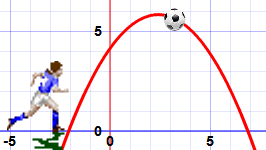## Standard Form

The Standard Form of a Quadratic Equation looks like this:• ab and c are known values. a can’t be 0.
• x” is the variable or unknown (we don’t know it yet).

Here are some examples:

 2x2 + 5x + 3 = 0 In this one a=2, b=5 and c=3 x2 − 3x = 0 This one is a little more tricky:Where is a? Well a=1, as we don’t usually write “1x2“b = −3And where is c? Well c=0, so is not shown. 5x − 3 = 0 So This one is not a quadratic equation: it is missing x2 (in other words a=0, which means it can’t be quadratic)

As we saw before, the Standard Form of a Quadratic Equation is

ax2 + bx + c = 0

But sometimes a quadratic equation doesn’t look like that!

For example:

In disguise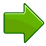In Standard Forma, b and c
x2 = 3x − 1Move all terms to left hand sidex2 − 3x + 1 = 0a=1, b=−3, c=1
2(w2 − 2w) = 5Expand (undo the brackets),
and move 5 to left
2w2 − 4w − 5 = 0a=2, b=−4, c=−5
z(z−1) = 3Expand, and move 3 to leftz2 − z − 3 = 0a=1, b=−1, c=−3

### Plus/Minus

First of all what is that plus/minus thing that looks like ± ?

The ± means there are TWO answers:

x = −b + √(b− 4ac)2a

x = −b − √(b− 4ac)2a

Here is an example with two answers:But it does not always work out like that!

• Imagine if the curve “just touches” the x-axis.
• Or imagine the curve is so high it doesn’t even cross the x-axis!

This is where the “Discriminant” helps us …

### Discriminant

Do you see b2 − 4ac in the formula above? It is called the Discriminant, because it can “discriminate” between the possible types of answer:

• when b2 − 4ac is positive, we get two Real solutions
• when it is zero we get just ONE real solution (both answers are the same)
• when it is negative we get a pair of Complex solutions

Complex solutions? Let’s talk about them after we see how to use the formula.

Just put the values of a, b and c into the Quadratic Formula, and do the calculations.

### Example: Solve 5x2 + 6x + 1 = 0

Coefficients are:a = 5, b = 6, c = 1
Quadratic Formula:x = −b ± √(b− 4ac)2a
Put in a, b and c:x = −6 ± √(6− 4×5×1)2×5
Solve:x = −6 ± √(36 − 20)10
x = −6 ± √(16)10
x = −6 ± 410
x = −0.2 or −1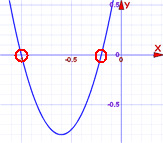Answer: x = −0.2 or x = −1

And we see them on this graph.

 Check -0.2: 5×(−0.2)2 + 6×(−0.2) + 1 = 5×(0.04) + 6×(−0.2) + 1 = 0.2 − 1.2 + 1 = 0 Check -1: 5×(−1)2 + 6×(−1) + 1 = 5×(1) + 6×(−1) + 1 = 5 − 6 + 1 = 0

### Remembering The Formula

A kind reader suggested singing it to “Pop Goes the Weasel”:

 ♫ “x is equal to minus b ♫ “All around the mulberry bush plus or minus the square root The monkey chased the weasel of b-squared minus four a c The monkey thought ’twas all in fun ALL over two a” Pop! goes the weasel”

Try singing it a few times and it will get stuck in your head!

Or you can remember this story:

x = −b ± √(b− 4ac)2a

“A negative boy was thinking yes or no about going to a party,
at the party he talked to a square boy but not to the 4 awesome chicks.
It was all over at 2 am.

## Complex Solutions?

When the Discriminant (the value b2 − 4ac) is negative we get a pair of Complex solutions … what does that mean?

It means our answer will include Imaginary Numbers. Wow!

### Example: Solve 5x2 + 2x + 1 = 0

Coefficients are:a=5, b=2, c=1
Note that the Discriminant is negative:b2 − 4ac = 22 − 4×5×1
−16
Use the Quadratic Formula:x = −2 ± √(−16)10

√(−16) = 4i
(where i is the imaginary number √−1)

So:x = −2 ± 4i10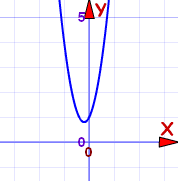Answer: x = −0.2 ± 0.4i

The graph does not cross the x-axis. That is why we ended up with complex numbers.

In some ways it is easier: we don’t need more calculation, just leave it as −0.2 ± 0.4i.

### Example: Solve x2 − 4x + 6.25 = 0

Coefficients are:a=1, b=−4, c=6.25
Note that the Discriminant is negative:b2 − 4ac = (−4)2 − 4×1×6.25
−9
Use the Quadratic Formula:x = −(−4) ± √(−9)2

√(−9) = 3i
(where i is the imaginary number √−1)

So:x = 4 ± 3i2Answer: x = 2 ± 1.5i

The graph does not cross the x-axis. That is why we ended up with complex numbers.BUT an upside-down mirror image of our equation does cross the x-axis at 2 ± 1.5 (note: missing the i).

Just an interesting fact for you!

## Summary

• Quadratic Equation in Standard Form: ax2 + bx + c = 0
• Quadratic Equations can be factored
• Quadratic Formula: x = −b ± √(b− 4ac)2a
• When the Discriminant (b2−4ac) is:
• positive, there are 2 real solutions
• zero, there is one real solution
• negative, there are 2 complex solutions

More examples of factoring quadratics with a leading coefficient of 1 | Algebra II | Khan Academy

#### Attachments32

• Missing Attachment
• Missing Attachment
• Missing Attachment
• Missing Attachment
• Missing Attachment
• Missing Attachment
• Missing Attachment
• Missing Attachment
• Missing Attachment
• Missing Attachment
• Missing Attachment
• Missing Attachment
• Missing Attachment
• Missing Attachment
• Missing Attachment
• Missing Attachment
• Missing Attachment
• Missing Attachment
• Missing Attachment
• Missing Attachment
• Missing Attachment
• Missing Attachment
• Missing Attachment
• Missing Attachment
• Missing Attachment
• Missing Attachment
• Missing Attachment
• Missing Attachment
• Missing Attachment
• Missing Attachment
• Missing Attachment
• Missing Attachment